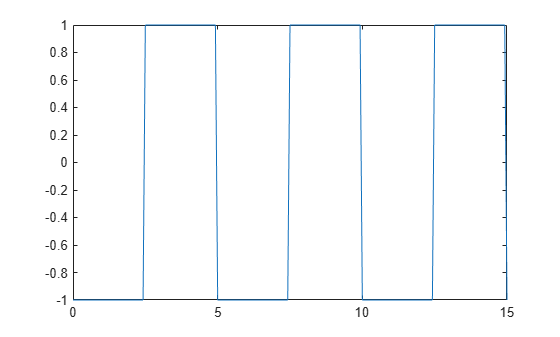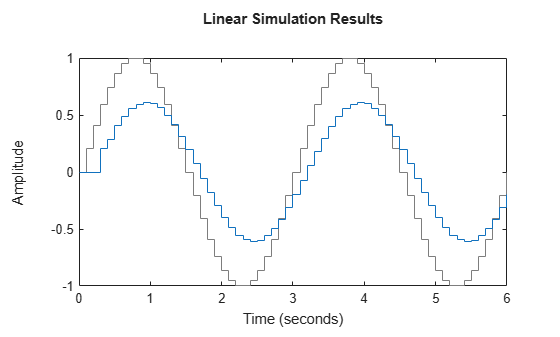# gensig

Create periodic signals for simulating system response with `lsim`

## Syntax

``[u,t] = gensig(type,tau)``
``[u,t] = gensig(type,tau,Tf)``
``[u,t] = gensig(type,tau,Tf,Ts)``

## Description

example

````[u,t] = gensig(type,tau)` generates a unit-amplitude periodic signal with the specified type and period. Use the signal `u` and corresponding time vector `t` to simulate the time response of a single-input dynamic system using `lsim` or `lsimplot` or to obtain response characteristics using `lsiminfo`. To create signals for multi-input systems, use repeated calls to `gensig` and stack the resulting `u` vectors into a matrix. When you use `u` and `t` to simulate a dynamic system model, the software interprets `t` as having the units of the `TimeUnit` property of the model.```

example

````[u,t] = gensig(type,tau,Tf)` generates a signal with a duration of `Tf`. `t` runs from 0 to `Tf` in increments of `tau/64`.```

example

````[u,t] = gensig(type,tau,Tf,Ts)` generates a signal with a sample time of `Ts`. `t` runs from 0 to `Tf` in increments of `Ts`. To generate a signal for simulating a discrete-time model, use this syntax and set `Ts` to the sample time of the model.```

## Examples

collapse all

Generate a square wave with a period of 2 s to use for simulating a dynamic system response with `lsim`.

```tau = 2; [u,t] = gensig("square",tau);```

`gensig` returns the signal as the vector `u` and the corresponding time vector `t`. When you do not specify the duration of the signal, `gensig` generates a signal that runs for five periods (`Tf = 5*tau`). When you do not specify a time step, the function defaults to 64 samples per period (`Ts = tau/64`). Thus, this signal runs for 10 s with a time step of 0.03125 s. Plot the signal.

`plot(t,u)``gensig` returns a square wave of unit amplitude that starts at zero. You can modify `u` to obtain a square wave with a different amplitude and different endpoints. Create a square wave of period 5 that runs for 15 s, and that switches between values of –1 and 1.

```tau = 5; Tf = 15; [u0,t] = gensig("square",tau,Tf); u = 2*u0-1; plot(t,u)```Use `t` and `u` to simulate the response of a dynamic system with `lsim`. The `lsim` command assumes the values of `t` are in the units of the dynamic system model that you simulate (`sys.TimeUnit`).

```sys = tf(30,[1 5 30]); lsim(sys,u,t)```If you do not specify a time step (sample time), `gensig` defaults to 64 samples per period, or `Ts = tau/64`. When you want to simulate a discrete-time model with `lsim`, the time step must equal the sample time of the model. Provide `gensig` with this sample time to generate a suitable signal. For example, generate a sine wave for simulating a discrete-time dynamic system model with a 0.1 s sample time.

```tau = 3; Tf = 6; Ts = 0.1; [u,t] = gensig("sine",tau,Tf,Ts);```

Simulate the model response to the generated signal.

```sys = zpk([],[-0.1,-0.5],1,Ts); lsim(sys,u,t,Ts)```To simulate a multi-input system with `lsim`, you provide the input signals as a matrix whose columns represent the signal applied to each input. In other words, `u(:,j)` is the signal applied to the `j`th input at each time step. To use `gensig` to generate such an input matrix, create the signals for each input together and stack them together in a matrix.

For instance, create a signal for simulating a two-input system that injects a square wave of period 2 s into the first input, and a pulse every 1.5 s into the second input. Specify a duration and sample time so that the two vectors have the same length, which is necessary for combining them into a matrix.

```Tf = 8; Ts = 0.02; [uSq,t] = gensig("square",2,Tf,Ts); [uPu,~] = gensig("pulse",1.5,Tf,Ts); u = [uSq uPu]; size(u)```
```ans = 1×2 401 2 ```

Each row `u(i,:)` of `u` is the signal `(u1,u2)` applied to the inputs at the corresponding time `t(i)`.

You can combine signals that are not created with `gensig` provided they have the same length. For instance, suppose that you want to simulate a three-input system by applying `uSq` to the first input and `uPu` to second input. You also want to apply a ramp to the third input that starts at 0 and increases to 1 at the final time `Tf = 8`. Ensure that the signal is a column vector with the same length as `uSq` and `uPu`. Then combine it with the other signals to create the input matrix.

```uRa = linspace(0,1,401)'; u = [uSq uPu uRa]; size(u)```
```ans = 1×2 401 3 ```
`plot(t,u)`You can now use `u` and `t` to simulate a three-input model. Generate a three-input, two-output state-space model, and simulate the response at its two outputs to `u` applied at the inputs.

```rng('default') sys = rss(3,2,3); lsim(sys,u,t)```## Input Arguments

collapse all

Type of periodic signal to generate, specified as one of the following:

• `"sine"` — Sine wave

• `"square"` — Square wave

• `"pulse"` — Periodic pulse

All signals have unit amplitude and have the initial value 0 at ```t = 0```. You can specify the type with a character vector instead of a string (for example, `'sine'`).

Period of generated signal, specified as a positive scalar value. Specify `tau` in the units of the dynamic system model you want to simulate with `lsim`. For instance, if `sys.TimeUnit` is `'seconds'`, then to generate a signal for simulating `sys` with a period of 30 s, set `tau` to 30. If `sys.TimeUnit` is `'minutes'`, then to generate such a signal, set `tau` to 0.5.

Duration of signal for simulation, specified as a positive scalar value. The output vector `t` is of the form `0:Ts:Tf`, where the time step is set by `Ts`. When you use `t` with `lsim` to simulate a dynamic system model, `lsim` interprets `t` as having the units specified in the `TimeUnit` property of the model.

Time step, specified as a positive scalar value. The output vector `t` is of the form `0:Ts:Tf`. The units of `Ts` are the units specified by the `TimeUnit` property dynamic system model you intend to simulate with `lsim`. When you are simulating a discrete-time model, set `Ts` equal to the sample time `Ts` of the model.

## Output Arguments

collapse all

Generated signal, returned as a column vector of the same length as `t`. The shape of the signal is determined by `type`. The signal has unit amplitude with a baseline of 0.

Time vector, returned as a column vector of the form `0:Ts:Tf`. If you do not specify a duration `Tf`, then `gensig` uses `Tf = 5*``tau`. If you do not specify a time step `Ts`, then `gensig` uses `tau/64`.

## Version History

Introduced before R2006a